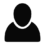Select Page

# Reply To: how to script sums of A/D data

Optuma Forums Optuma Scripting how to script sums of A/D data Reply To: how to script sums of A/D data

#55319

Hi David,

Do you have the data sets for the Advances and Declines?

Assuming that the first two lines get the respective data files (I can not remember what they are) then this should work.

dec1 = GetData(code={decline ticker});

// now we need to accumulate the values
accdec = ACC(dec1, bars=10);

// now we can do the division

If you don’t have the tickers then we can do it in the breadth engine instead. Let us know and we can update a script for that.

All the best

Mathew

###### 1 user thanked author for this post.Andy